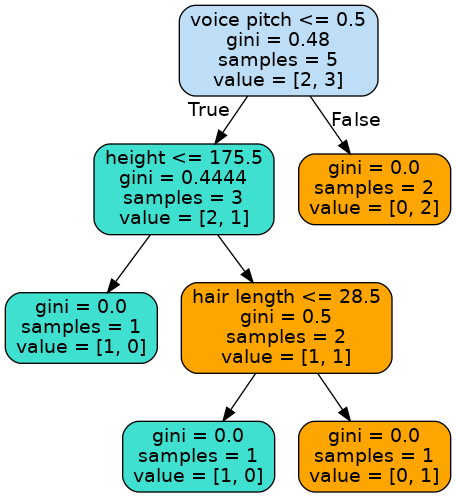Decision tree is one of the simplest and most popular classification algorthms to learn, understand, and interpret. In is often utilized to deal with classification and regression problems.

Related course:

### Decision tree

The decision tree algorithm is based from the concept of a decision tree which involves using a tree structure that is similar to a flowchart.It uses the following symbols:

• an internal node representing feature or attribute

• branch representing the decision rule, and leaf node representing the outcome.

• The highest node in the decision tree is called the root node; it is the one responsible in partitioning the tree in a recursive manner, also known as recursive partitioning.

This particular structure of the decision tree provides a good visualization that helps in decision making and problem solving.

It is similar to a flowchart diagram that mimics the way human beings think. This is what makes decision trees easy to understand and interpret.

Its also easy in code:

### Advantages of Using a Decision Tree for Classification

Aside from its simplicity and ease of interpretation, here are the other advantaged of using decision tree fo classification in machine learning.

1. Considered a white box type of ML algorithm, decision tree uses an internal decision-making logic; this means that the acquired knowledge from a data set can be easily extracted in a readable form  which is not a feature of black box algorithms such as Neural Network. This makes the training time of decision tree faster compared to the latter.

2. Due to its simplicty, anyone can code, visualize, interpret, and manipulate simple decision trees, such as the naive binary type. Even for beginners, the decision tree classifier is easy to learn and understand. It requires its users minimal effort for data preparation and analysis.

3. The decision tree follows a non-parametric method; meaning, it is distribution-free and does not depend on probability distribution assumptions. It can work on high-dimensional data with excellent accuracy.

4. Decision trees can perform feature selection or variable screening completely. They can work on both caregorical and numerical data. Furthermore, they can handle problems with multiple results or outputs.

5. Unlike other classification algorithm, when you use decision trees, nonlinear relationships between parameters do not influence the trees performance.

## How the Decision Tree Algorithm Works

To understand these advantages more clearly, let us discuss what the basic idea behind the decision tree algorithm is.

1. Choose the best or most appropriate attribute using Attribute Selection Measures(ASM) in order to divide the records.
2. Transform that attribute to become a decision node and divide the dataset to create smaller subsets.
3. Start to create trees by repeating the process recursively for each child. When it matches one of the conditions below, the process shall end:
• All the tuples are contained in the same attribute value.
• No more attributes remain.
• No more instances.